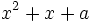# Galois extensions for cyclic group:Z2

View Galois extensions for particular groups | View other specific information about cyclic group:Z2

Given a Galois extension of fields, the Galois group for the extension is the cyclic group of order two if and only if the field extension is a quadratic extension.

## Over a finite field

Over a finite field, there is exactly one quadratic extension.

### Case where the field does not have characteristic two

In this case, the quadratic extension can be obtained by adjoining a polynomial of the form$x^2 - a$ where$a$ is a nonzero element of the field that is not a square. For prime fields, such an element is termed a quadratic nonresidue.

Since the multiplicative group of a finite field is cyclic, the set of quadratic nonresidues is exactly half the number of nonzero elements (this can also be seen more directly, for instance, by noting that exactly half the numbers of squares because every number has two squareroots). Thus, exactly half the polynomials$x^2 - a$, for$a$ nonzero, are irreducible.

### Case where the field has characteristic two

This case is a little trickier, since no polynomial of the form$x^2 - a$ is irreducible. Rather, an irreducible polynomial is of the form$x^2 + x + a$.

For instance, for the field of two elements, the polynomial$x^2 + x + 1$ is irreducible.